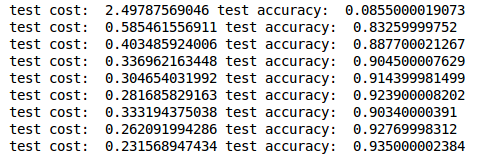# RNN Classifier 循环神经网络

## 方法介绍 ¶

from keras.layers import SimpleRNN, Activation, Dense


MNIST里面的图像分辨率是28×28，为了使用RNN，我们将图像理解为序列化数据。 每一行作为一个输入单元，所以输入数据大小INPUT_SIZE = 28； 先是第1行输入，再是第2行，第3行，第4行，…，第28行输入， 这就是一张图片也就是一个序列，所以步长TIME_STEPS = 28

X_train = X_train.reshape(-1, 28, 28) / 255.      # normalize
X_test = X_test.reshape(-1, 28, 28) / 255.        # normalize


## 搭建模型 ¶

model.add(SimpleRNN(
# for batch_input_shape, if using tensorflow as the backend, we have to put None for the batch_size.
# Otherwise, model.evaluate() will get error.
batch_input_shape=(None, TIME_STEPS, INPUT_SIZE),
output_dim=CELL_SIZE,
unroll=True,
))


model.add(Dense(OUTPUT_SIZE))


## 训练 ¶

for step in range(4001):
# data shape = (batch_num, steps, inputs/outputs)
X_batch = X_train[BATCH_INDEX: BATCH_INDEX+BATCH_SIZE, :, :]
Y_batch = y_train[BATCH_INDEX: BATCH_INDEX+BATCH_SIZE, :]
cost = model.train_on_batch(X_batch, Y_batch)
BATCH_INDEX += BATCH_SIZE
BATCH_INDEX = 0 if BATCH_INDEX >= X_train.shape else BATCH_INDEX


if step % 500 == 0:
cost, accuracy = model.evaluate(X_test, y_test, batch_size=y_test.shape, verbose=False)
print('test cost: ', cost, 'test accuracy: ', accuracy)